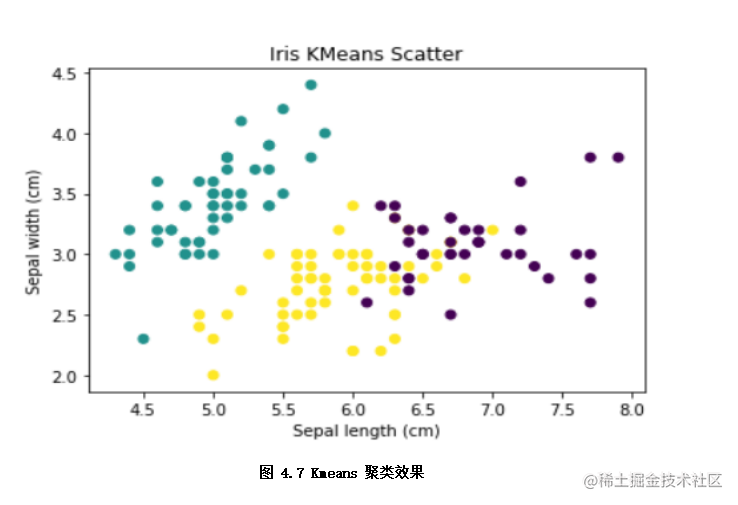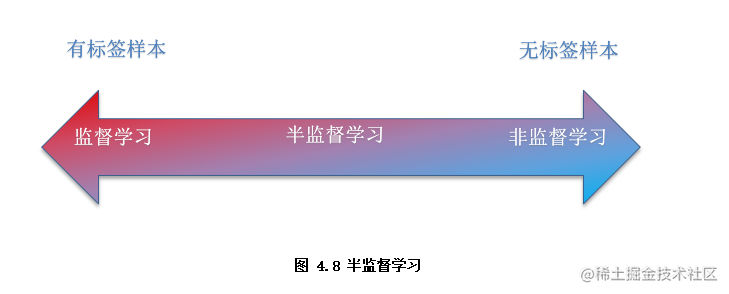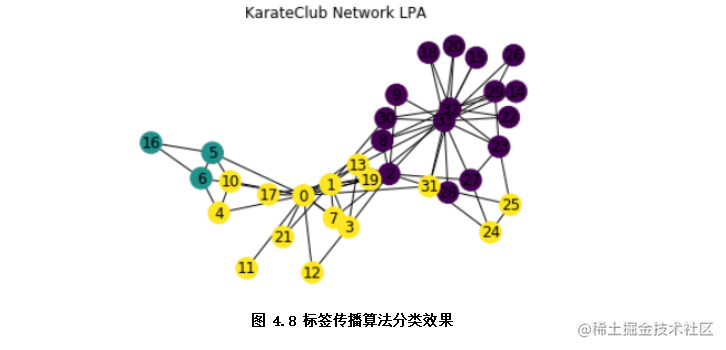# 一文速览机器学习的类别（Python代码）

## 一、 机器学习类别

### 1.1 监督学习

• 逻辑回归简介

。其中模型参数w即对应各特征（x1, x2, x3...）的权重（w1,w2,w3...），b模型参数代表着偏置项，Y为预测结果（0~1范围）。

• 代码示例

``````import pandas as pd    # 导入pandas库

from sklearn.linear_model import LogisticRegression  # 导入逻辑回归模型
x = weather_df.drop('If Rain', axis=1)  # 特征x
y = weather_df['If Rain']  # 标签y
lr = LogisticRegression()
lr.fit(x, y)  # 模型训练
print("前10个样本预测结果：", lr.predict(x[0:10]) ) # 模型预测前10个样本并输出结果

• 线性回归简介

• 代码示例
``````from sklearn.linear_model import LinearRegression  #导入线性回归模型
x = weather_df.drop('Humidity', axis=1)  # 特征x
y = weather_df['Humidity']  # 标签y
linear = LinearRegression()

linear.fit(x, y)  # 模型训练
print("前10个样本预测结果：", linear.predict(x[0:10]) ) # 模型预测前10个样本并输出结果
# 前10个样本预测结果： [0.42053525 0.32811401 0.31466161 0.3238797  0.29984453 0.29880059

### 1.2 非监督学习

• Kmeans聚类简介

Kmeans聚类是非监督学习常用的方法，其原理是先初始化k个簇类中心，通过迭代算法更新各簇类样本，实现样本与其归属的簇类中心的距离最小的目标。其算法步骤为： 1.初始化：随机选择 k 个样本作为初始簇类中心（可以凭先验知识、验证法确定k的取值）； 2.针对数据集中每个样本 计算它到 k 个簇类中心的距离，并将其归属到距离最小的簇类中心所对应的类中； 3.针对每个簇类 ，重新计算它的簇类中心位置； 4.重复上面 2 、3 两步操作，直到达到某个中止条件（如迭代次数，簇类中心位置不变等）

• 代码示例
``````from sklearn.datasets import load_iris  # 数据集
from sklearn.cluster import KMeans   #  Kmeans模型
import matplotlib.pyplot as plt  # plt画图
x = lris_df.data
k = 3  # 聚类出k个簇类, 已知数据集有三类品种, 设定为3
model = KMeans(n_clusters=k)
model.fit(x)  # 训练模型
print("前10个样本聚类结果：",model.predict(x[0:10]) ) # 模型预测前10个样本并输出聚类结果：[1 1 1 1 1 1 1 1 1 1]
# 样本的聚类效果以散点图展示
x_axis = lris_df.data[:,0] # 以iris花的sepal length (cm)特征作为x轴
y_axis = lris_df.data[:,1] # 以iris花的sepal width (cm)特征作为y轴
plt.scatter(x_axis, y_axis, c=model.predict(x)) # 分标签颜色展示聚类效果
plt.xlabel('Sepal length (cm)')#设定x轴注释
plt.ylabel('Sepal width (cm)')#设定y轴注释
plt.title('Iris KMeans Scatter')
plt.show()   # 如图4.7聚类效果### 1.3半监督学习• 标签传播算法简介

1. 首先利用样本间的关系（可以是样本客观关系，或者利用相似度函数计算样本间的关系）建立完全图模型。

2. 接着向图中加入已标记的标签信息（或无），无标签节点是用一个随机的唯一的标签初始化。

3. 将一个节点的标签设置为该节点的相邻节点中出现频率最高的标签，重复迭代，直到标签不变即算法收敛。

• 代码示例

``````import networkx as nx # 导入networkx图网络库
import matplotlib.pyplot as plt
from networkx.algorithms import community  # 图社区算法
G=nx.karate_club_graph()   # 加载美国空手道俱乐部图数据
#注： 本例未使用已标记信息, 严格来说是半监督算法的无监督应用案例
lpa = community.label_propagation_communities(G)  # 运行标签传播算法
community_index = {n: i for i, com in enumerate(lpa) for n in com} # 各标签对应的节点
node_color = [community_index[n] for n in G]  # 以标签作为节点颜色
pos = nx.spring_layout(G)  # 节点的布局为spring型
nx.draw_networkx_labels(G, pos) # 节点序号
nx.draw(G, pos, node_color=node_color) # 分标签颜色展示图网络
plt.title(' Karate_club network LPA')
plt.show() #展示分类效果，不同颜色为不同类别